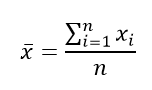Jet Set Go! All about Aeroplanes Jet Set Go! All about Aeroplanes

# Sample Statistic

A sample statistic is any quantity from the sample of a population. A sample is a group of elements chosen from the population. The features that describe the population are called the parameters and the properties of the sample data are known as statistics. Population and sample both are important parts of statistics.

Inferential statistics gives methods to generalize the population characteristics by using the sample statistics. Suppose a report reads, “50% of kindergarten children in India watch TV for more than 20 hrs per week”. This conclusion is not arrived at by surveying the TV watching habits of all kindergarten children in India. On the contrary, this conclusion would have been arrived at by making an estimate of the population parameters using sample space. It is not generally practical to find the population parameters by studying all the elements of the population. In most cases, the population parameters are generalized using statistics of representative and unbiased samples.

## What is a Sample in Statistics?

A sample statistic is a numerical descriptive measure of a sample. A statistic is usually derived from measurements of the individuals in the sample. The statistics are a characteristic of a sample data distribution like mean, median, mode, standard deviation and proportions. A sample statistic could be the measure of any characteristic of the sample.

### Sample Statistic Symbol

The following table shows different notations used for parameters and statistics:

 Characteristic Sample Statistic Population Parameter Mean x̄ μ Standard deviation s σ Proportion p^ p

The sample size is indicated by the lowercase letter ‘n’ and the population size by the uppercase letter ‘N’.

## Sample Statistic Formula

Let us see here the formulas for sample statistics for mean and standard deviation.

### Sample Mean Formula

Computing the sample mean is not different from computing the population mean. We add all the data values and divide this sum by the number of values in the data set. Therefore, the sample mean is;### Sample Standard Deviation Formula

The standard deviation of the population is estimated using the formula √(∑(xi−x̄)2/n) to compute the standard deviation of a small sample that underestimates the population parameter.

In order to get an unbiased estimate of the population standard deviation, the n in the numerator is replaced by n – 1. Therefore,

Sample Standard Deviation = √(∑(xi−x̄)2/n-1)

### Population Parameter in Sample Statistic

A measure found from analyzing sample data is a sample statistic. An important aspect of inferential statistics attempts to estimate population parameters using sample statistics. The mean of an unbiased sample collected using random methods can be used as an estimator of the mean of the population which is represented by the sample and the population is approximately normal.

Specifically, if the sample mean x̄ is used as the estimate of the population mean μ, we say that x̄ is a point estimate of the population mean μ. The sample mean x̄ is the best estimator of the population mean μ than the sample median and sample mode. The reason is the means of many samples of the same population vary less than the median and mode of the different samples.

Properties of Estimators:

1. The estimator should be unbiased. This means that the mean of estimates obtained from different samples of the same size should be equal to the parameter being estimated.
2. The estimator should be consistent. The estimator should approach the parameter value as the sample size is increased.
3. The estimator should be efficient. Amongst the various estimators of a population parameter, the relatively efficient estimator should have the smallest variance.

### Example

The following GPA score of 30 High School students. Find the sample mean and standard deviation.

3.1, 2.9, 2.8, 2.9, 3.8, 4.8, 4.2, 3.9, 3.4, 2.5, 4.2, 3.7, 3.3, 2.1, 3.8, 3.0, 3.7, 4.0, 2.7, 3.8, 3.2, 3.5, 3.5, 3.6, 2.2, 3.1, 3.5, 4.0, 2.7, 4.5.

Solution: Using the formulas to compute the sample mean and standard deviation, we get,

Sample mean:

x̄ =∑x/n = 102.4/30 = 3.41

Sample standard deviation:

S = √(∑(xi−x̄)2/n-1) = 0.65.

## Frequently Asked Questions – FAQs

### What is the formula for Sample Mean?

The sample mean formula is given by adding all the data values and dividing this sum by the number of values in the data set.

### What is the standard deviation?

The standard deviation is a statistic that measures the dispersion of a dataset relative to its mean and is calculated as the square root of the variance.

### What is the standard variance?

Variance is the average of all data points within a group.

### Which is better standard deviation or variance?

The standard deviation is usually more useful to describe the variability of the data while the variance is usually much more useful mathematically.

### Why is standard deviation important?

Standard deviation is important because it tells us how spread out the values are in a given dataset.
Test your knowledge on Sample Statistic